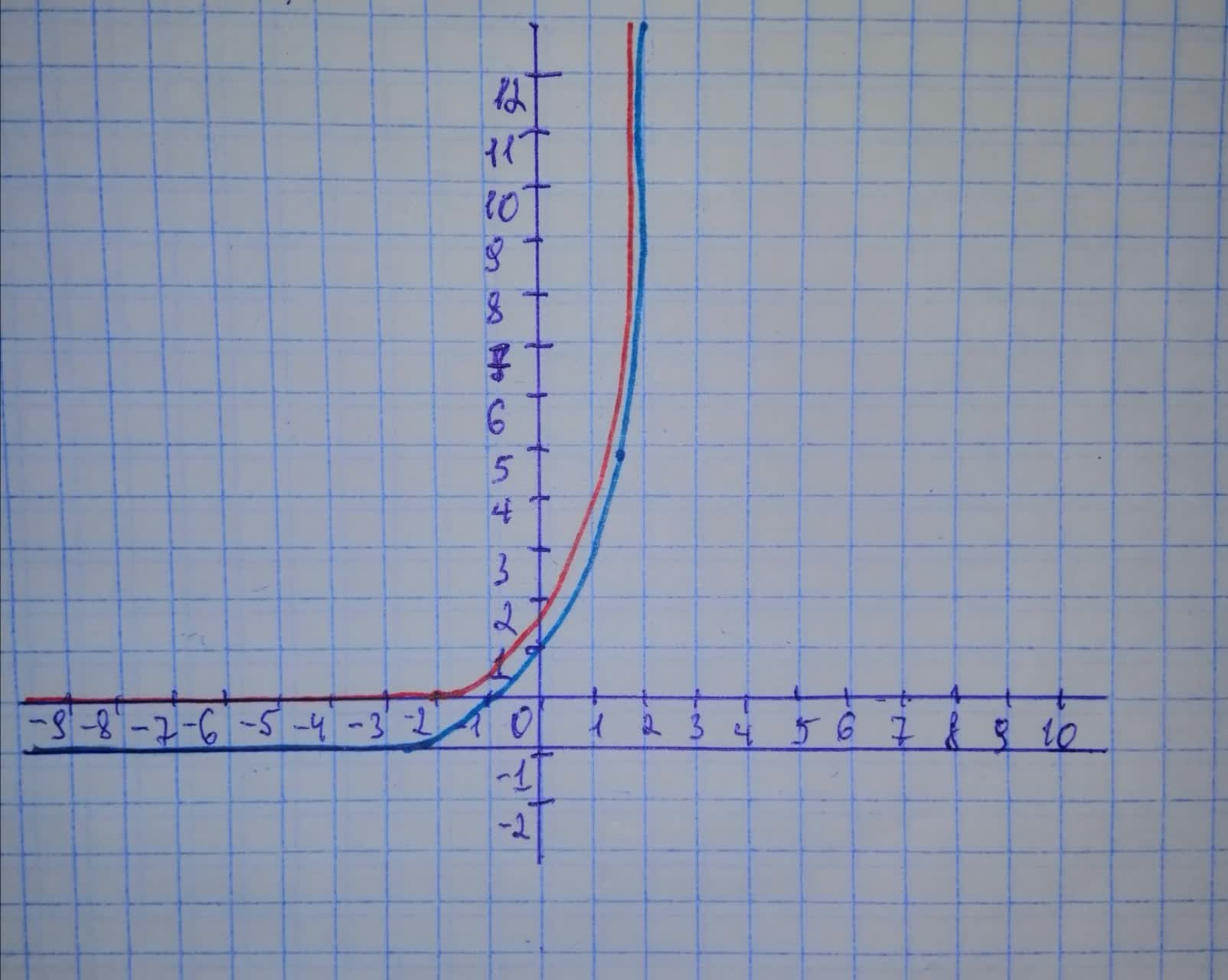Question# Graph f and g in the same rectangular coordinate system. Use transformations of the graph of f to obtain the graph of g. Graph and give equations of all asymptotes. Use the graphs to determine each function's domain and range. f(x)=3^{x}=3^{x} - 1

Transformations of functions
ANSWEREDGraph f and g in the same rectangular coordinate system. Use transformations of the graph of f to obtain the graph of g. Graph and give equations of all asymptotes. Use the graphs to determine each function's domain and range. $$\displaystyle{f{{\left({x}\right)}}}={3}^{{{x}}}={3}^{{{x}}}\ -\ {1}$$2021-02-11

$$\displaystyle{f{{\left({x}\right)}}}={3}^{{{x}}}\ {\quad\text{and}\quad}\ {g{{\left({x}\right)}}}={3}^{{{x}}}\ -\ {1}$$ Graph of g(x) can be obtained by translating the graph of f(x) by 1 unit downwards along the y-axis. $$\displaystyle\text{Domain of}\ {f{{\left({x}\right)}}}\ {i}{s}\ {x}\ \in\ {\left(-\infty,\ \infty\right)}$$
$$\displaystyle\text{Range of}{f{{\left({x}\right)}}}\ {i}{s}\ {\left({0},\ \infty\right)}$$
$$\displaystyle\text{Asymptote for the graph is}\ {y}={0}$$
$$\displaystyle\text{Domain of}\ {g{{\left({x}\right)}}}\ {i}{s}\ {x}\ \in\ {\left(-\infty,\ \infty\right)}$$
$$\displaystyle\text{Range of}{g{{\left({x}\right)}}}\ {i}{s}\ {\left(-{1},\ \infty\right)}$$
$$\displaystyle\text{Asymptote for the graph is}\ {y}=\ -{1}$$ g(x) is the PURPLE curve, and f(x) is the RED curve